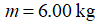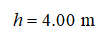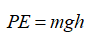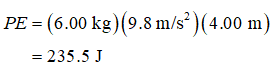# 39.) Given a box of mass 6.00 kg at a height of 4.00 meters above the floor. The box has gravitational potential energy relative to the floor of value PE. Making which of these changes would cause the gravitational potential energy of the box to be 4PE? a.change the mass of the box to 24.0 kg b.change the height of the box to 4.00 m

Question
1 views
39.) Given a box of mass 6.00 kg at a height of 4.00 meters above the floor. The box has gravitational potential energy relative to the floor of value PE. Making which of these changes would cause the gravitational potential energy of the box to be 4PE?
 a. change the mass of the box to 24.0 kg b. change the height of the box to 4.00 m
check_circle

Step 1

Mass of the box,Step 2

Height above the floor,Step 3

The gravitational potential energy relative to the floor,...

### Want to see the full answer?

See Solution

#### Want to see this answer and more?

Solutions are written by subject experts who are available 24/7. Questions are typically answered within 1 hour.*

See Solution
*Response times may vary by subject and question.
Tagged in